# Matplotlib Scatter Plot Color by Category in Python

Posted on Aug 30, 2020 · 6 mins read

Scatter plot are useful to analyze the data typically along two axis for a set of data. It shows the relationship between two sets of data

The data often contains multiple categorical variables and you may want to draw scatter plot with all the categories together

The coloring of each category in the scatter plot is important to visualize the relationship among different categories

In this post we will see how to color code the categories in a scatter plot using matplotlib and seaborn

## Scatter Plot Color by Category using Matplotlib

Matplotlib scatter has a parameter c which allows an array-like or a list of colors. The code below defines a `colors` dictionary to map your Continent colors to the plotting colors.

``````import matplotlib.pyplot as plt
import numpy as np
import pandas as pd

population = np.random.rand(100)
Area = np.random.randint(100,600,100)
continent =['North America','Europe', 'Asia', 'Australia']*25

df = pd.DataFrame(dict(population=population, Area=Area, continent = continent))

fig, ax = plt.subplots()

colors = {'North America':'red', 'Europe':'green', 'Asia':'blue', 'Australia':'yellow'}

ax.scatter(df['population'], df['Area'], c=df['continent'].map(colors))

plt.show()
``````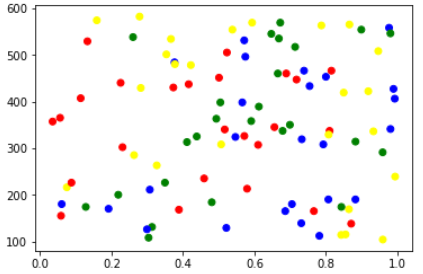## Color by Category using Seaborn

Seaborn has a scatter plot that shows relationship between `x` and `y` can be shown for different subsets of the data using the `hue`, `size`, and `style` parameters. These parameters control what visual semantics are used to identify the different subsets

The `hue` parameter is used for Grouping variable that will produce points with different colors. Can be either categorical or numeric, although color mapping will behave differently in latter case.

``````import seaborn as sns
sns.scatterplot('population', 'Area', data=df, hue='continent')

plt.show()
``````

Alternatively, we can also use lmplot function that combines `regplot()` and `FacetGrid`. It is intended as a convenient interface to fit regression models across conditional subsets of a dataset.

We will set the `fit_reg` parameter to False because we don’t want to estimate and plot a regression model relating the `x` and `y` variables

``````import seaborn as sns
sns.lmplot('population', 'Area', data=df, hue='continent', fit_reg=False)

plt.show()
``````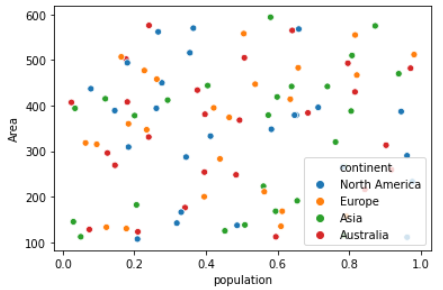## Color by Category using Pandas Groupby

We will loop over pandas grouped object(df.groupby) and create individual scatters and manually assign colors

This code assumes the same DataFrame as above and then groups it based on `color`. It then iterates over these groups, plotting for each one.

To select a color I’ve created a `colors` dictionary which can map the Continent color (for instance `North America`) to a real color (for instance `red`).

``````fig, ax = plt.subplots()

colors = {'North America':'red', 'Europe':'green', 'Asia':'blue', 'Australia':'yellow'}

grouped = df.groupby('continent')
for key, group in grouped:
group.plot(ax=ax, kind='scatter', x='population', y='Area', label=key, color=colors[key])

plt.show()
``````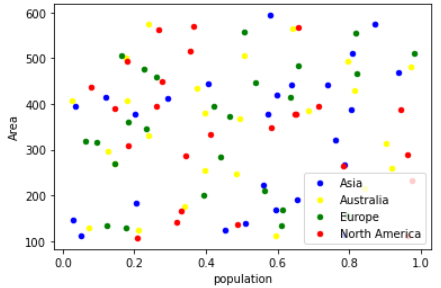## Seaborn Color Palette

This function provides an interface to many of the possible ways you can generate colors in seaborn. It return a list of colors defining a color palette.

It takes 2 parameters i.e. Name of Palette and Number of colors in the palette

We will create the color palette first

``````sns.palplot(sns.color_palette("muted"))
``````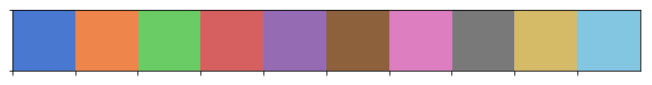And then map this color palette with the Color Labels i.e. Unique Continents in our data set

``````# Get Unique continents
color_labels = df['continent'].unique()

# List of colors in the color palettes
rgb_values = sns.color_palette("Set2", 4)

# Map continents to the colors
color_map = dict(zip(color_labels, rgb_values))

# Finally use the mapped values
plt.scatter(df['population'], df['Area'], c=df['continent'].map(color_map))
``````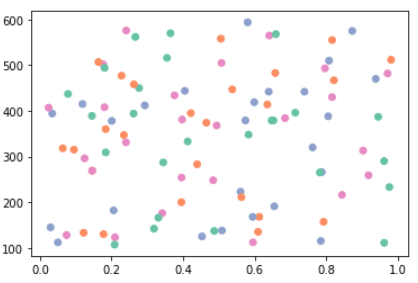## Matplotlib Colormap

Colormap instances are used to convert data values (floats) from the interval `[0, 1]` to the RGBA color that the respective Colormap represents

With this scatter plot we can visualize the different dimension of the data: the x,y location corresponds to Population and Area, the size of point is related to the total population and color is related to particular continent

``````rng = np.random.RandomState(0)
x = df['population']
y = df['Area']
colors = {'North America':'red', 'Europe':'green', 'Asia':'blue', 'Australia':'yellow'}

plt.scatter(x, y, s=100*df['population'], alpha=0.3,
c= df['continent'].map(colors),
cmap='viridis')
plt.colorbar();
``````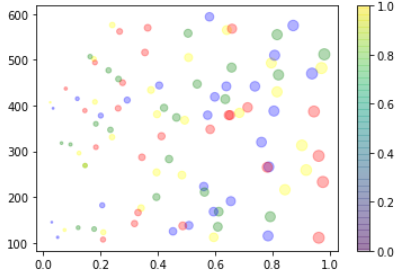Multicolor and multifeature scatter plots like this can be useful for both exploration and presentation of data